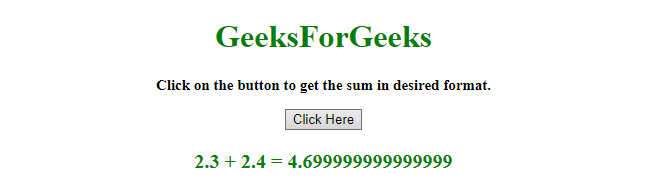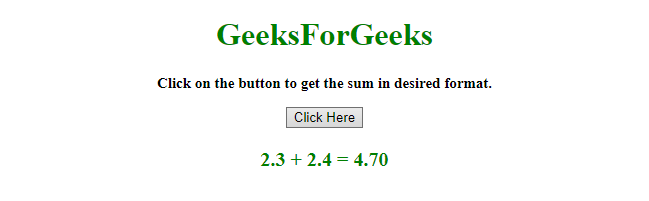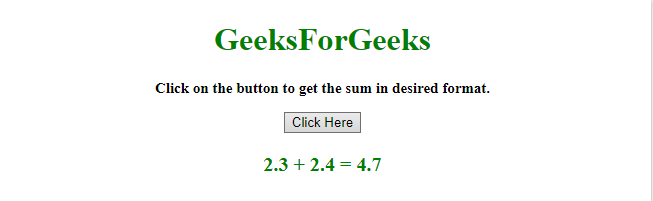# How to add float numbers using JavaScript ?

• Last Updated : 11 Dec, 2019

Given two or more numbers and the task is to get the float addition in the desired format with the help of JavaScript. There are two methods to solve this problem which are discussed below:

Approach 1:

Hey geek! The constant emerging technologies in the world of web development always keeps the excitement for this subject through the roof. But before you tackle the big projects, we suggest you start by learning the basics. Kickstart your web development journey by learning JS concepts with our JavaScript Course. Now at it's lowest price ever!

Example: This example implements the above approach.

 `  ` `<``html``>  ` ` `  `<``head``>  ` `    ``<``title``>  ` `        ``Float sum with JavaScript. ` `    `` ` `  ` ` `  `<``body` `style` `= ``"text-align:center;"``>  ` `     `  `    ``<``h1` `style` `= ``"color:green;"` `>  ` `        ``GeeksForGeeks  ` `    `` ` `     `  `    ``<``p` `id` `= ``"GFG_UP"` `style` `= ` `        ``"font-size: 15px; font-weight: bold;"``> ` `    `` ` `     `  `    ``<``button` `onclick` `= ``"gfg_Run()"``>  ` `        ``Click Here ` `    `` ` `     `  `    ``<``p` `id` `= ``"GFG_DOWN"` `style = "color:green; ` `        ``font-size: 20px; font-weight: bold;"> ` `    `` ` `     `  `    ``<``script``> ` `        ``var el_up = document.getElementById("GFG_UP"); ` `        ``var el_down = document.getElementById("GFG_DOWN"); ` `         `  `        ``el_up.innerHTML = "Click on the button to get the" ` `                          ``+ " sum in desired format."; ` `         `  `        ``var val = parseFloat('2.3')+parseFloat('2.4'); ` `        ``el_down.innerHTML = "2.3 + 2.4 = " + val; ` `         `  `        ``function gfg_Run() { ` `            ``el_down.innerHTML = "2.3 + 2.4 = "  ` `                        ``+ (parseFloat('2.3') +  ` `                        ``parseFloat('2.4')).toFixed(2); ` `        ``}  ` `    ``  ` `  ` ` `  ` `

Output:

• Before clicking on the button:• After clicking on the button:Approach 2:

Example: This example implements the above approach.

 `  ` `<``html``>  ` ` `  `<``head``>  ` `    ``<``title``>  ` `        ``Float sum with javascript. ` `    `` ` `  ` ` `  `<``body` `style` `= ``"text-align:center;"``>  ` `     `  `    ``<``h1` `style` `= ``"color:green;"` `>  ` `        ``GeeksForGeeks  ` `    `` ` `     `  `    ``<``p` `id` `= ``"GFG_UP"` `style` `= ` `        ``"font-size: 15px; font-weight: bold;"``> ` `    `` ` `     `  `    ``<``button` `onclick` `= ``"gfg_Run()"``>  ` `        ``Click Here ` `    `` ` `     `  `    ``<``p` `id` `= ``"GFG_DOWN"` `style = "color:green; ` `        ``font-size: 20px; font-weight: bold;"> ` `    `` ` `     `  `    ``<``script``> ` `        ``var el_up = document.getElementById("GFG_UP"); ` `        ``var el_down = document.getElementById("GFG_DOWN"); ` `         `  `        ``el_up.innerHTML = "Click on the button to get the" ` `                          ``+ " sum in desired format."; ` `         `  `        ``var val = parseFloat('2.3')+parseFloat('2.4'); ` `        ``el_down.innerHTML = "2.3 + 2.4 = " + val; ` `         `  `        ``function gfg_Run() { ` `            ``el_down.innerHTML = "2.3 + 2.4 = " +  ` `                    ``Math.round((parseFloat('2.3') ` `                    ``+ parseFloat('2.4'))*100)/100; ` `        ``}  ` `    ``  ` `  ` ` `  ` `

Output:

• Before clicking on the button:• After clicking on the button:My Personal Notes arrow_drop_up
Recommended Articles
Page :